# Ant on a rubber rope facts for kids

Kids Encyclopedia Facts

The ant on a rubber rope is a mathematical puzzle with a solution that appears counterintuitive or paradoxical. It is sometimes given as a worm, or inchworm, on a rubber or elastic band, but the principles of the puzzle remain the same.

The details of the puzzle can vary, but a typical form is as follows:

An ant starts to crawl along a taut rubber rope 1 km long at a speed of 1 cm per second (relative to the rubber it is crawling on). At the same time, the rope starts to stretch uniformly at a constant rate of 1 km per second, so that after 1 second it is 2 km long, after 2 seconds it is 3 km long, etc. Will the ant ever reach the end of the rope?

At first consideration it seems that the ant will never reach the end of the rope, but in fact it does. (In the form stated above, it would take 8.9×1043421 years.) Whatever the length of the rope and the relative speeds of the ant and the stretching, provided that the ant's speed and the stretching remain steady, the ant will always be able to reach the end given sufficient time. Once the ant has begun moving, the rubber rope is stretching both in front of and behind the ant, conserving the proportion of the rope already walked by the ant and enabling the ant to make continual progress.

An ant (red dot) crawling on a stretchable rope at a constant speed of 1 cm/s. The rope is initially 4 cm long and stretches at a constant rate of 2 cm/s.

## A formal statement of the problem

The problem as stated above requires some assumptions to be made. The following fuller statement of the problem attempts to make most of those assumptions explicit. The informal statements like the one given in the introduction of this article are obtained by simplifying the following statement and assigning values to the variables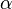$\alpha$ and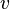$v$.

Consider a thin and infinitely stretchable rubber rope held taut along an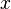$x$-axis with a starting point marked at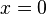$x=0$ and a target point marked at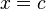$x=c$, with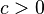$c>0$.
At time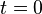$t=0$ the rope starts to stretch uniformly and smoothly in such a way that the starting point remains stationary at$x=0$ while the target point moves away from the starting point with constant speed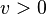$v>0$.
A small ant leaves the starting point at time$t=0$ and walks steadily and smoothly along the rope towards the target point at a constant speed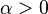$\alpha>0$ relative to the point on the rope where the ant is at each moment.
Will the ant reach the target point?

## Solutions of the problem

### A discrete mathematics solution

Although solving the problem appears to require analytical techniques, it can actually be answered by a combinatorial argument by considering a variation in which the rope stretches suddenly and instantaneously each second rather than stretching continuously. Indeed, the problem is sometimes stated in these terms, and the following argument is a generalisation of one set out by Martin Gardner, originally in Scientific American and later reprinted.

Consider a variation in which the rope stretches suddenly and instantaneously before each second, so that the target-point moves from$x=c$ to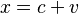$x=c+v$ at time$t=0$, and from$x=c+v$ to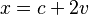$x=c+2v$ at time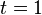$t=1$, etc. Many versions of the problem have the rope stretch at the end of each second, but by having the rope stretch before each second we have disadvantaged the ant in its goal, so we can be sure that if the ant can reach the target point in this variation then it certainly can in the original problem, or indeed in variants where the rope stretches at the end of each second.

Let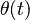$\theta(t)$ be the proportion of the distance from the starting-point to the target point which the ant has covered at time t. So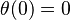$\theta(0)=0$. In the first second the ant travels distance$\alpha$, which is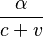$\frac{\alpha}{c+v}$ of the distance from the starting-point to the target-point (which is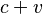$c+v$ throughout the first second). When the rope stretches suddenly and instantaneously,$\theta(t)$ remains unchanged, because the ant moves along with the rubber where it is at that moment. So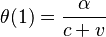$\theta(1)=\frac{\alpha}{c+v}$. In the next second the ant travels distance$\alpha$ again, which is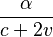$\frac{\alpha}{c+2v}$ of the distance from the starting-point to the target-point (which is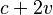$c+2v$ throughout that second). So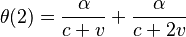$\theta(2)=\frac{\alpha}{c+v}+\frac{\alpha}{c+2v}$. Similarly, for any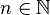$n\in\mathbb{N}$,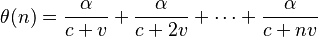$\theta(n)=\frac{\alpha}{c+v}+\frac{\alpha}{c+2v}+\cdots+\frac{\alpha}{c+nv}$.

Notice that for any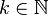$k\in\mathbb{N}$,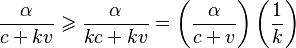$\frac{\alpha}{c+kv}\geqslant\frac{\alpha}{kc+kv}=\left(\frac{\alpha}{c+v}\right)\left(\frac{1}{k}\right)$, so we can write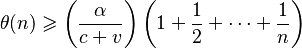$\theta(n)\geqslant\left(\frac{\alpha}{c+v}\right)\left(1+\frac{1}{2}+\cdots+\frac{1}{n}\right)$.

The term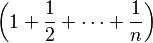$\left(1+\frac{1}{2}+\cdots+\frac{1}{n}\right)$ is a partial harmonic series, which diverges, so we can find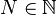$N\in\mathbb{N}$ such that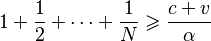$1+\frac{1}{2}+\cdots+\frac{1}{N}\geqslant\frac{c+v}{\alpha}$, which means that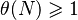$\theta(N)\geqslant1$.

Therefore, given sufficient time, the ant will complete the journey to the target point. This solution could be used to obtain an upper bound for the time required, but does not give an exact answer for the time it will take.

### An analytical solution

A key observation is that the speed of the ant at a given time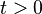$t>0$ is its speed relative to the rope, i.e.$\alpha$, plus the speed of the rope at the point where the ant is. The target-point moves with speed$v$, so at time$t$ it is at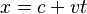$x=c+vt$. Other points along the rope move with proportional speed, so at time$t$ the point on the rope at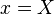$x=X$ is moving with speed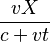$\frac{vX}{c+vt}$. So if we write the position of the ant at time$t$ as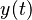$y(t)$, and the speed of the ant at time$t$ as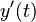$y'(t)$, we can write: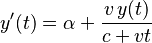$y'(t)=\alpha+\frac{v\,y(t)}{c+vt}$

This is a first order linear differential equation, and it can be solved with standard methods. However, to do so requires some moderately advanced calculus. A much simpler approach considers the ant's position as a proportion of the distance from the starting-point to the target-point.

Consider coordinates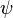$\psi$ measured along the rope with the starting-point at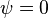$\psi=0$ and the target-point at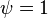$\psi=1$. In these coordinates, all points on the rope remain at a fixed position (in terms of$\psi$) as the rope stretches. At time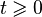$t\geqslant0$, a point at$x=X$ is at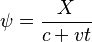$\psi=\frac{X}{c+vt}$, and a speed of$\alpha$ relative to the rope in terms of$x$, is equivalent to a speed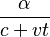$\frac{\alpha}{c+vt}$ in terms of$\psi$. So if we write the position of the ant in terms of$\psi$ at time$t$ as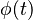$\phi(t)$, and the speed of the ant in terms of$\psi$ at time$t$ as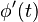$\phi'(t)$, we can write: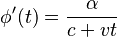$\phi'(t)=\frac{\alpha}{c+vt}$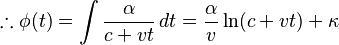$\therefore\phi(t)=\int{\frac{\alpha}{c+vt}\,dt}=\frac{\alpha}{v}\ln(c+vt)+\kappa$ where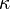$\kappa$ is a constant of integration.

Now,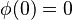$\phi(0)=0$ which gives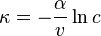$\kappa=-\frac{\alpha}{v}\ln{c}$, so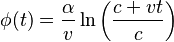$\phi(t)=\frac{\alpha}{v}\ln{\left(\frac{c+vt}{c}\right)}$.

If the ant reaches the target-point (which is at$\psi=1$) at time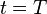$t=T$, we must have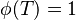$\phi(T)=1$ which gives us: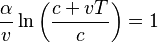$\frac{\alpha}{v}\ln{\left(\frac{c+vT}{c}\right)}=1$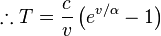$\therefore T=\frac{c}{v}\left(e^{v/\alpha}-1\right)$

(For the simple case of v=0, we can consider the limit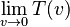$\lim_{v \rightarrow 0} T(v)$ and obtain the simple solution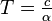$T= \tfrac{c}{\alpha}$ ) As this gives a finite value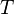$T$ for all finite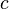$c$,$v$,$\alpha$ ($v>0$,$\alpha>0$), this means that, given sufficient time, the ant will complete the journey to the target-point. This formula can be used to find out how much time is required.

For the problem as originally stated,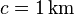$c=1\,\mathrm{km}$,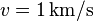$v=1\,\mathrm{km}/\mathrm{s}$ and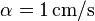$\alpha=1\,\mathrm{cm}/\mathrm{s}$, which gives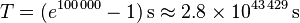$T=(e^{100\,000}-1)\,\mathrm{s}\,\!\approx2.8\times10^{43\,429}\,\mathrm{s}$. This is a vast timespan, even compared to the estimated age of the universe, which is only about 4×1017 s. Furthermore, the length of the rope after such a time is similarly huge, so it is only in a mathematical sense that the ant can ever reach the end of this particular rope.

## Intuition

Regardless of the speed of the endpoint of the rope, we can always make marks on the rope so that the relative speed of any two adjacent marks is arbitrarily slow. If the rope initially is 1 km long and is stretched by 1 km per second, we can make marks that are initially 5 mm apart along the whole rope. The relative speed of any two marks is then 5 mm per second. It is obvious that an ant crawling at 1 cm per second always can get from one mark to the next, and then to the next again and so on, until it eventually reaches the end of the rope. The same reasoning works for any constant stretching speeds, ant speeds and rope lengths.

The key fact is that the ant moves together with the points of the rope when the rope is being stretched. At any given point of time we can find the proportion of the distance from the starting-point to the target-point which the ant has covered. Even if the ant stops and the rope continues to be stretched, this proportion will not decrease and will in fact remain constant as the ant travels together with the point on the rope where the ant stopped (because the rope is stretched uniformly). Therefore, if the ant moves forward this proportion is only going to increase.

## Metric expansion of space

This puzzle has a bearing on the question of whether light from distant galaxies can ever reach us given the metric expansion of space. The universe is expanding, which leads to increasing distances to other galaxies, and galaxies that are far enough away from us will have an apparent relative motion greater than the speed of light. It might seem that light leaving such a distant galaxy could never reach us.

By thinking of photons of light as ants crawling along the rubber rope of space between the galaxy and us, we can see that just as the ant can eventually reach the end of the rope, so light from distant galaxies, even some that appear to be receding at a speed greater than the speed of light, can eventually reach Earth, given sufficient time.

However, the metric expansion of space is accelerating. An ant on a rubber rope whose expansion increases with time is not guaranteed to reach the endpoint. The light from sufficiently distant galaxies may still therefore never reach Earth.Ant on a rubber rope Facts for Kids. Kiddle Encyclopedia.